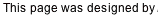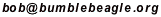## Du-Sum-Oh Solving Tutorial

Below is the puzzle we want to solve. We need to place the letters A-H in the cells so that each row contains exactly one of each letter. Ditto for each column and for each region. Several letters have been given to start us off.

This tutorial refers to rows and columns by number, and to regions by the starter letter in the region.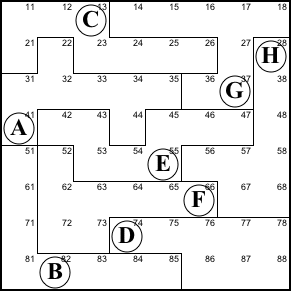Step 1. Rows 1, 2, and 3 share the 22 purple cells below left with regions A, C, and G. The columns have the to extra pink cells, while the regions have the two extra blue cells. Both the regions and the rows have three full sets of letters. So whatever is in the two pink cells must be the same as what's in the two blue cells. So 44 is H and 38 is A.

This the big law of leftovers. Wherever a group of regions overlaps some rows or columns, the parts outside the overlap (the leftovers) have to be the same.

44=H, 38=A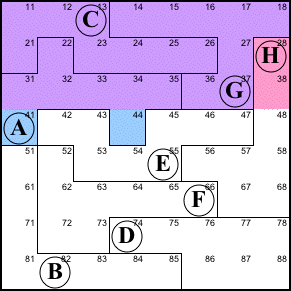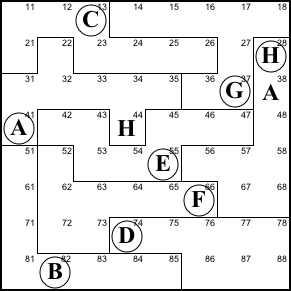Step 2. Using the big law of leftovers again, we see that 22 is G and 36 is H.

22=G, 36=H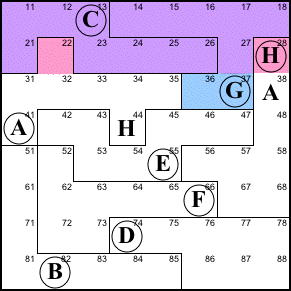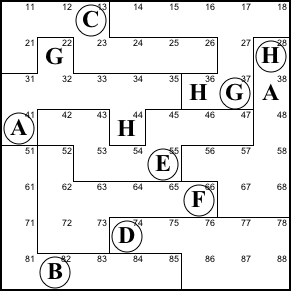Step 3. The little law of leftovers is a special case of the big law, applied to a single region and row/column. Here we see the blue and pink cells must both contain C, H, and G. 27 is C, and the G at 22 forces the pink G to 11 and H to 12.

27=C, 11=G, 12=H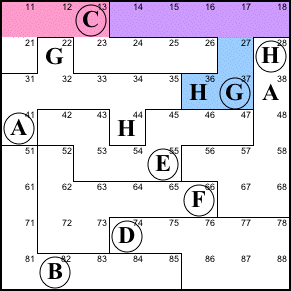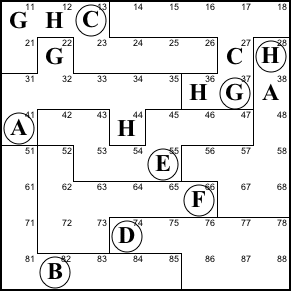Step 4. The big law of leftovers shows that 48 is E, 53 is H (because the H at 44 prevents 54 from being H) and 54 is A.

48=E, 53=H, 54=A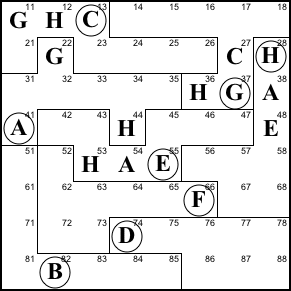Step 5. Region F lacks an H. Columns 2, 3, and 4 already have H, so the only place remaining is 65.

65=H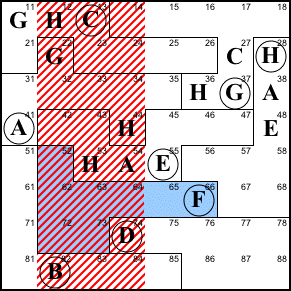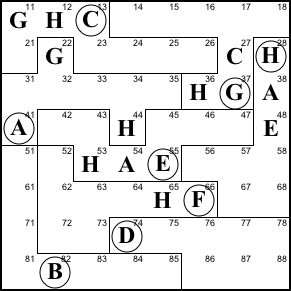Step 6. This is the most complicated step in the solution. The big law below, top left) shows us that 51 and 52 contain the same thing as 67 and 68, without indicating which matches up with which.

The B at 82 prevents either 51 or 52 from being B (below, top right). Thus neither 67 nor 68 can be B.

67 cannot be A, B, C, E, F, G, or H (below, bottom left), because its region already contains A, E, and H, its column contains C and G, its row contains F, and we just established it can't be B. Thus it has to be D.

67=D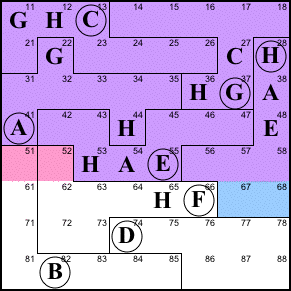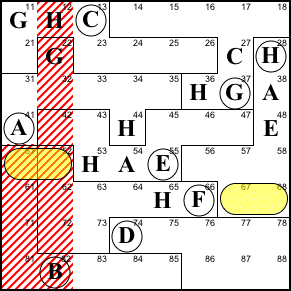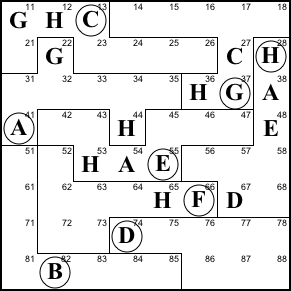Step 7. Neither 51 nor 52 can be a G because of the two Gs in columns 1 and 2. So 68 can't be G and for reasons similar to step 6, the only thing it can be is C.

68=C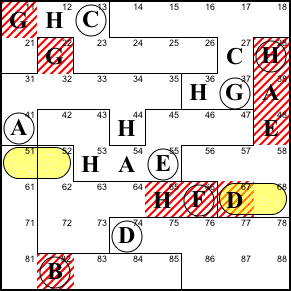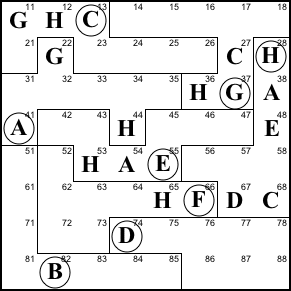Step 8. The only place in region F that can still be D is 52. And since 51+52=67+68, 51 must be C.

52=D, 51=C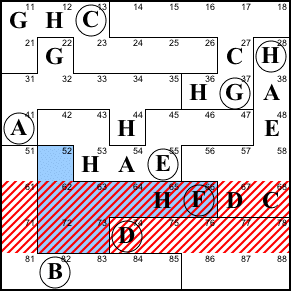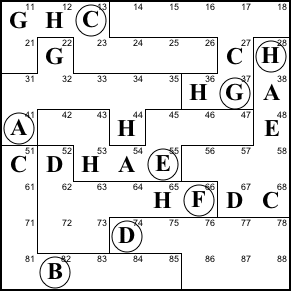Step 9. The little law says one of the blue cells must be C. But columns 7 and 8 already have C, so the C must be in 86.

86=C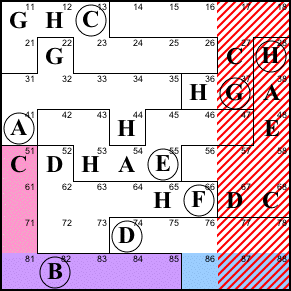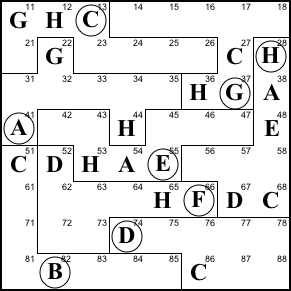Step 10. Row 7 needs a C, but 71 can't be C because of the C in region B; 73 can't be C due to the C in column 3; and 74 thru 78 are killed by the C in region D. So 72 is C.

This gives us 6 of the 8 Cs. The remaining two have to be at 34 and 45.

72=C, 45=C, 34=C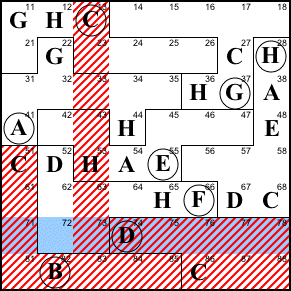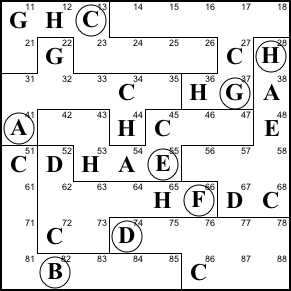Step 11. Column 2 lacks A, E, and F. Row 4 already contains A and E, so 42 is F. Then the A in row 3 means 32 is E, and 62 is A.

42=F, 32=E, 62=A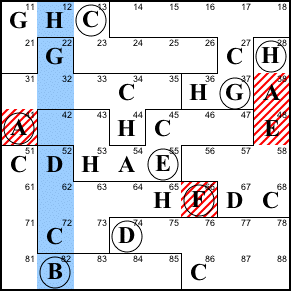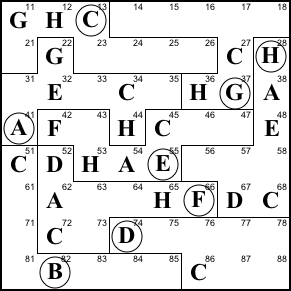Step 12. The only place left for D in column 1 is 18.

18=D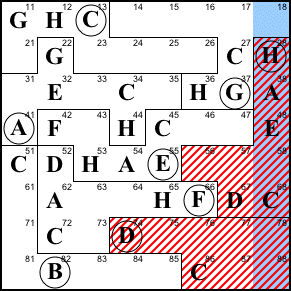Step 13. The only possibility for 61 is E.

61=E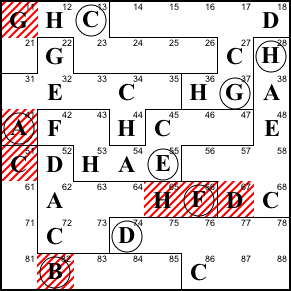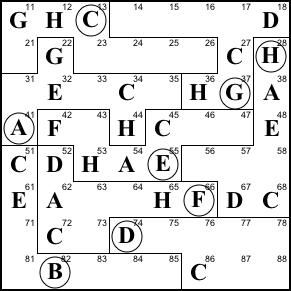Step 14. Column 7 lacks A, B, E, F, and H. As a member of row 4 and region E, 47 cannot be A, E, F, nor H, so it must be B. 57 can't be A, E, nor H (row 5) so it must be F.

The little law says 51+61+71 = 86+87+88. So 87 or 88 must be an E. But column 8 already has an E, so the E is 87.

That leave the A and H. 17 can't be H because region G already has H. So 17 is A and H is at 77.

47=B, 57=F, 87=E, 17=A, 77=H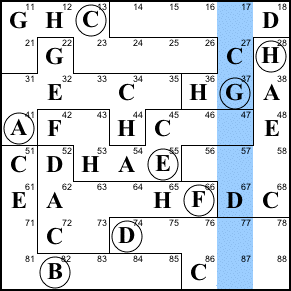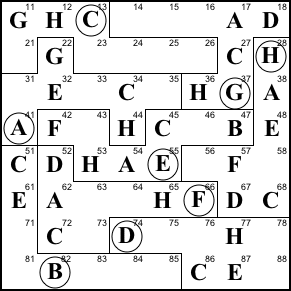Step 15. We've place 7 Hs, so the last one is at 81.

81=H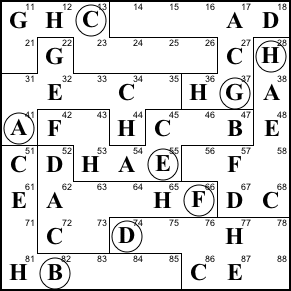Step 16. 71 can only be F. And the little law (51+61+71 = 86+87+88) says 88 is also F.

71=F, 88=F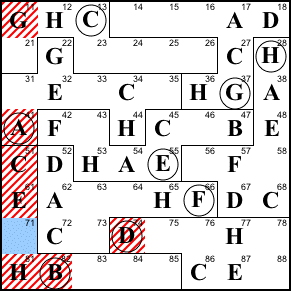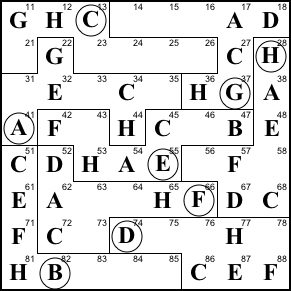Step 17. 84 can only be G.

84=G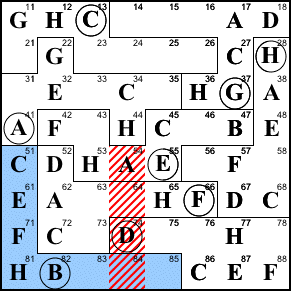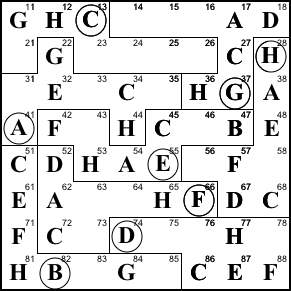Step 18. We can finish off region F by filling in B, E, and G in the only possible spots.

64=B, 63=G, 73=E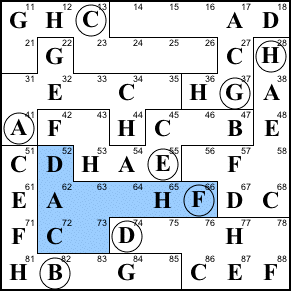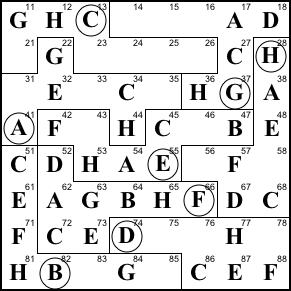Step 19. Finish row 4.

43=D, 46=G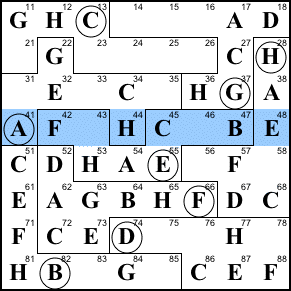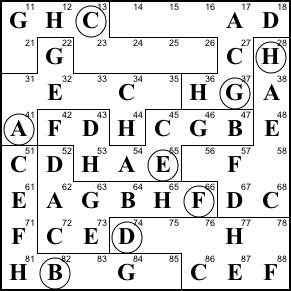Step 20. Finish regions B and H.

56=B, 58=G, 83=A, 85=D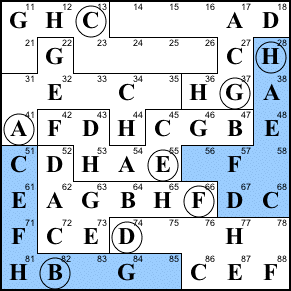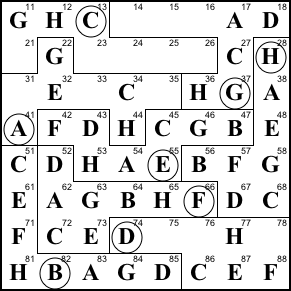Step 21. Finish row 7 and region G.

16=E, 14=F, 15=B, 78=B, 76=A, 75=G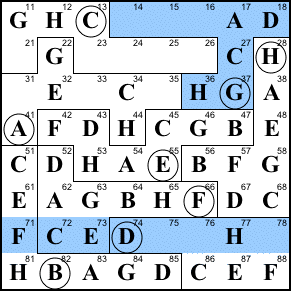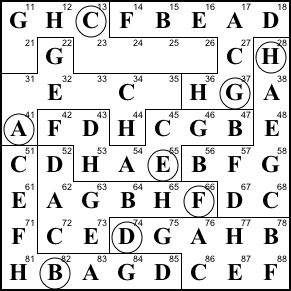Step 22. Finish column 6 and region A.

26=D, 35=F, 33=B, 31=D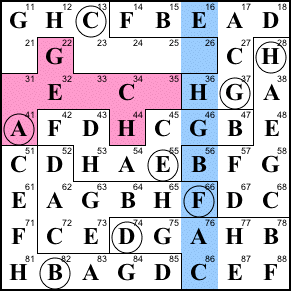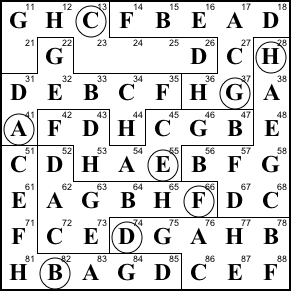Solution. Finish row 2, and the puzzle is complete.

21=B, 23=F, 24=E, 25=A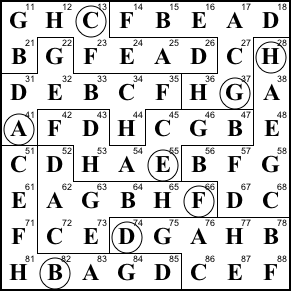Back to Bob’s main Du-Sum-Oh page.